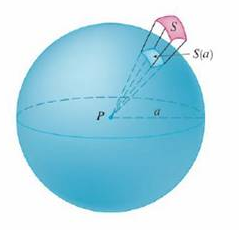Chapter 16, Problem 1P

Chapter
Section
Textbook Problem

1. Let S be a smooth parametric surface and let P be a point such that each line that starts at P intersects S at most once. The solid angle Ω(S) subtended by S at P is the set of lines starting at P and passing through S. Let S(a) be the intersection of Ω(S) with the surface of the sphere with center P and radius a. Then the measure of the solid angle (in steradians) is defined to be |Ω(S)| = area of   S ( a ) a 2 Apply the Divergence Theorem to the part of Ω(S) between S(a) and S to show that |Ω(S)| = ∬ S r · n r 3 dS where r is the radius vector from P to any point on S, r = | r |, and the unit normal vector n is directed away from P. This shows that the definition of the measure of a solid angle is independent of the radius a of the sphere. Thus the measure of the solid angle is equal to the area subtended on a unit sphere. (Note the analogy with the definition of radian measure.) The total solid angle subtended by a sphere at its center is thus 4π steradians.To determine

To show: The value of |Ω(S)|=Srnr3dS .

Explanation

Given data:

|Ω(S)|=areaofS(a)a2

Formula used:

Write the expression for Divergence Theorem.

EdivFdV=SFdS (1)

Consider the portion of Ω(S) and S is represented by S1 and S1 is the boundary.

Also consider the lateral surface of S1 is represented by SL .

Modify equation (1) as follows.

S1rnr3dS=S1rr3dV (2)

Consider r in terms of x , y and z .

r=xi+yj+zk

Consider r in terms of x , y and z represented as follows.

r=x2+y2+z2

Find the value of rr3 .

rr3=x,y,zx(x2+y2+z2)32,y(x2+y2+z2)32,z(x2+y2+z2)32=x[x(x2+y2+z2)32]+y[y(x2+y2+z2)32]+z[z(x2+y2+z2)32]=(x2+y2+z23x2)+(x2+y2+z23y2)+(x2+y2+z23z2)(x2+y2+z2)52=0

Substitute 0 for rr3 in equation (2),

S1rnr3dS=S1(0)dV=0

The surface of S1 other than S(a) and S is results in rn=0 .

Find the value of SLrnr3dS .

SLrnr3dS=SL0r3dS=0

Expand S1rnr3dS in terms of S(a) , S and SL as follows

Still sussing out bartleby?

Check out a sample textbook solution.

See a sample solution

The Solution to Your Study Problems

Bartleby provides explanations to thousands of textbook problems written by our experts, many with advanced degrees!

Get Started

Solve the equations in Exercises 126. x4x+1xx1=0

Finite Mathematics and Applied Calculus (MindTap Course List)

Evaluate the integral. 01(1+r)3dr

Single Variable Calculus: Early Transcendentals, Volume I

13. If , find n.

Mathematical Applications for the Management, Life, and Social Sciences

Sometimes, Always, or Never: If limxaf(x) and f(a) both exist, then f is continuous at a.

Study Guide for Stewart's Single Variable Calculus: Early Transcendentals, 8th